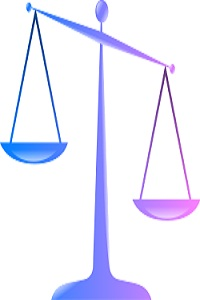# Chapter 8.2 Scales of Measurement

### Scales of MeasurementStatistical information, including numbers and sets of numbers, has specific qualities that are of interest to researchers.  These qualities, including magnitude, equal intervals, and absolute zero, determine what scale of measurement is being used and therefore what statistical procedures are best.  Magnitude refers to the ability to know if one score is greater than, equal to, or less than another score.  Equal intervals means that the possible scores are each an equal distance from each other.  And finally, absolute zero refers to a point where none of the scale exists or where a score of zero can be assigned.

When we combine these three scale qualities, we can determine that there are four scales of measurement.  The lowest level is the nominal scale, which represents only names and therefore has none of the three qualities.  A list of students in alphabetical order, a list of favorite cartoon characters, or the names on an organizational chart would all be classified as nominal data.  The second level, called ordinal data, has magnitude only, and can be looked at as any set of data that can be placed in order from greatest to lowest but where there is no absolute zero and no equal intervals.  Examples of this type of scale would include Likert Scales and the Thurstone Technique.

The third type of scale is called an interval scale, and possesses both magnitude and equal intervals, but no absolute zero.  Temperature is a classic example of an interval scale because we know that each degree is the same distance apart and we can easily tell if one temperature is greater than, equal to, or less than another.  Temperature, however, has no absolute zero because there is (theoretically) no point where temperature does not exist.

Finally, the fourth and highest scale of measurement is called a ratio scale.  A ratio scale contains all three qualities and is often the scale that statisticians prefer because the data can be more easily analyzed.  Age, height, weight, and scores on a 100-point test would all be examples of ratio scales.  If you are 20 years old, you not only know that you are older than someone who is 15 years old (magnitude) but you also know that you are five years older (equal intervals).  With a ratio scale, we also have a point where none of the scale exists; when a person is born his or her age is zero.

### Table 8.1: Scales of Measurement

Scale Level

Scale of Measurement

Scale Qualities

Example(s)

4

Ratio

Magnitude

Equal Intervals

Absolute Zero

Age, Height, Weight, Percentage

3

Interval

Magnitude

Equal Intervals

Temperature

2

Ordinal

Magnitude

Likert Scale, Anything rank ordered

1

Nominal

None

Names, Lists of words